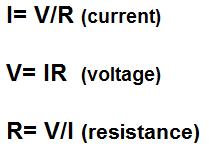﻿ Ohm's Law Calculator ﻿# Ohm's Law CalculatorEnter any 2 values for the 3rd value to be computed. Only 2 fields can be entered in for 3rd field to be computed.

 Voltage (V): V Current (I) A Resistance (R) Ω

nan

This ohm's law calculator calculates either the voltage, current, or resistance of a circuit, based on ohm's law.

Ohm's law can be represented in 3 different ways to calculate either the voltage, V, current, I, or resistance, R, of a circuit.

I=V/R is the the ohm's law formula used to calculate current in a circuit containing a resistor or linear resistive device.

V=IR is the ohm's law formula used to calculate the voltage in the circuit.

R=V/I is the ohm's law formula used to calculate the resistance in the circuit.

To use this calculator, the user just has to enter 2 of the 3 fields. For example, if the user wants to calculate the resistance of a linear circuit, he just enters in the voltage, V, and current, I, and clicks 'Calculate'. Resistance would then be computed. If a user wants to calculate the voltage of a circuit, he just enters in the current, I, and the resistance, R, leaving the voltage field blank. If a user wants to calculate the current of a circuit, he enters in the voltage, V, and the resistance, R, leaving the current field blank, and the current will be computed.

Ohm's Law is very useful in any circuits containing only linear resistive elements, such as resistors. It is not used with nonlinear components such as capacitors, inductors, diodes, or transistors.

Related Resources

﻿